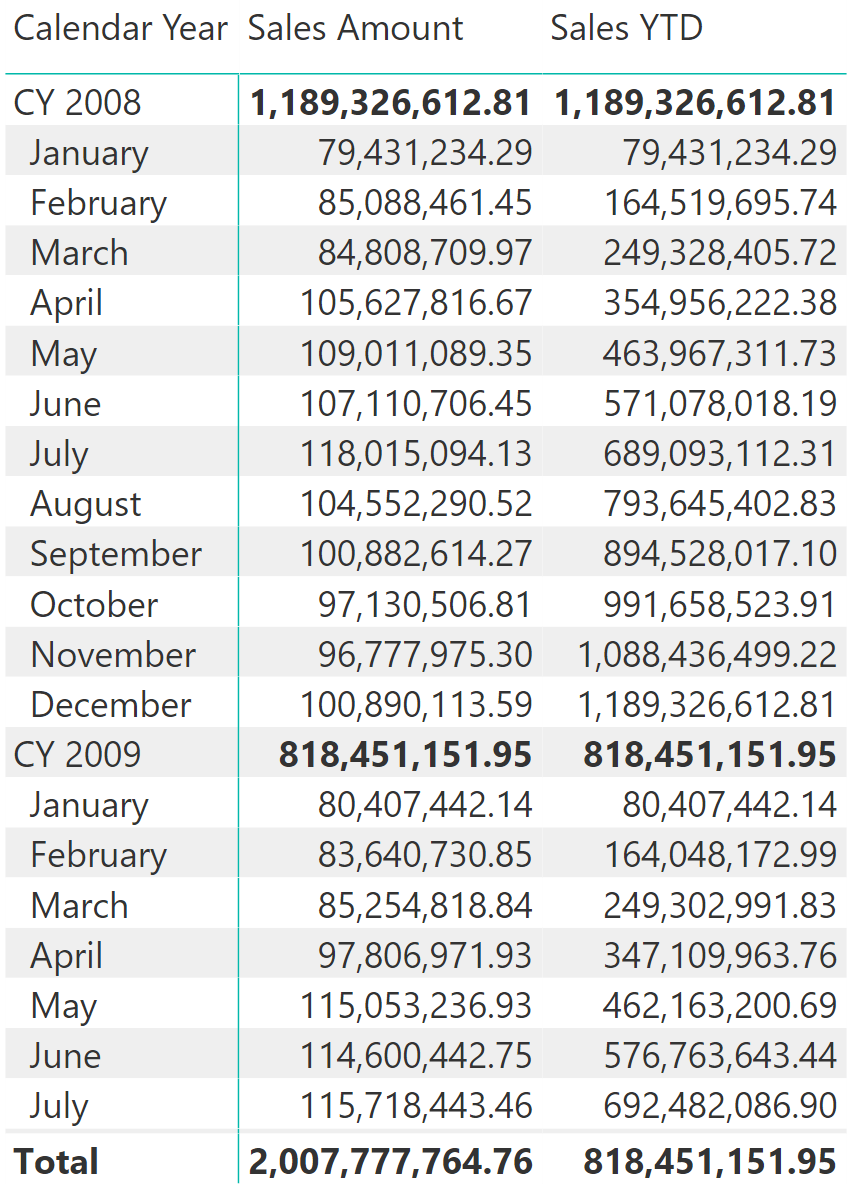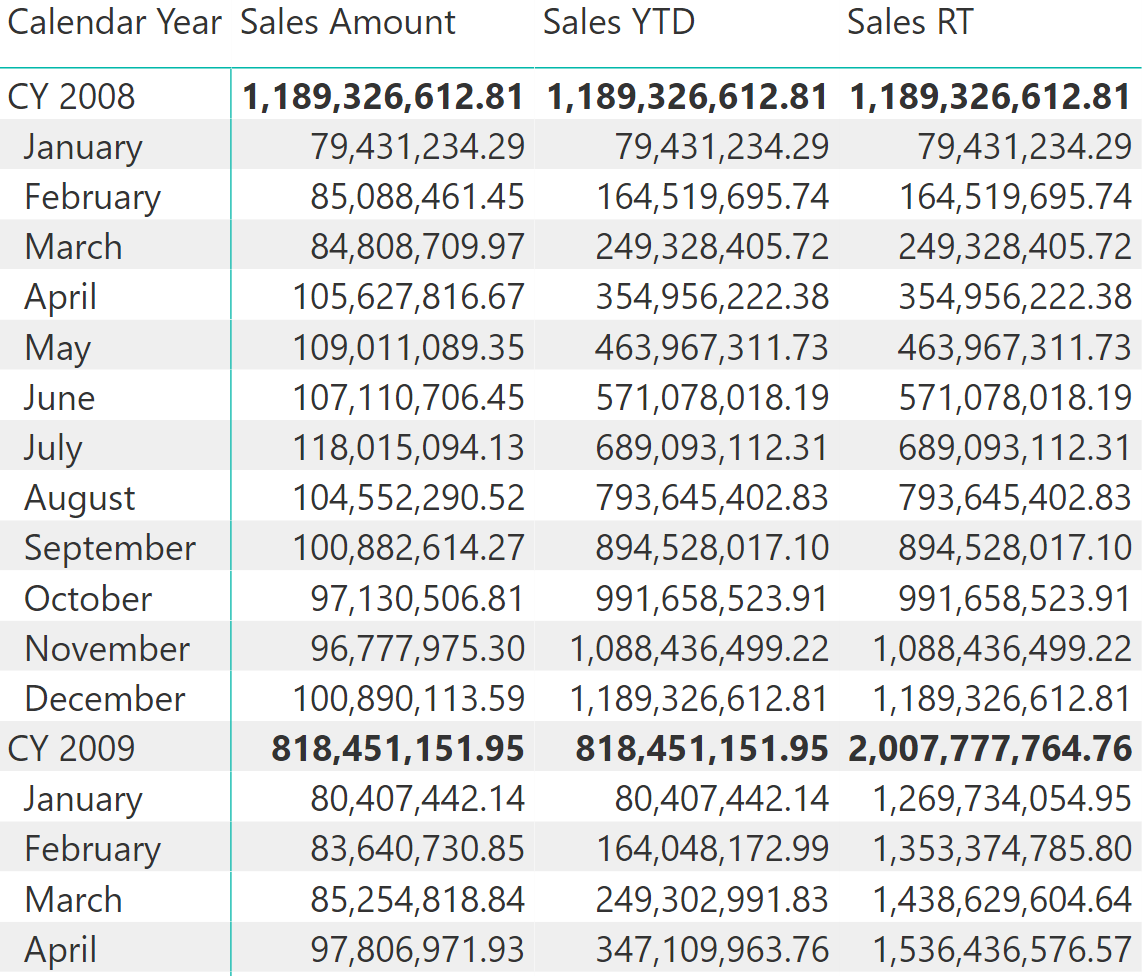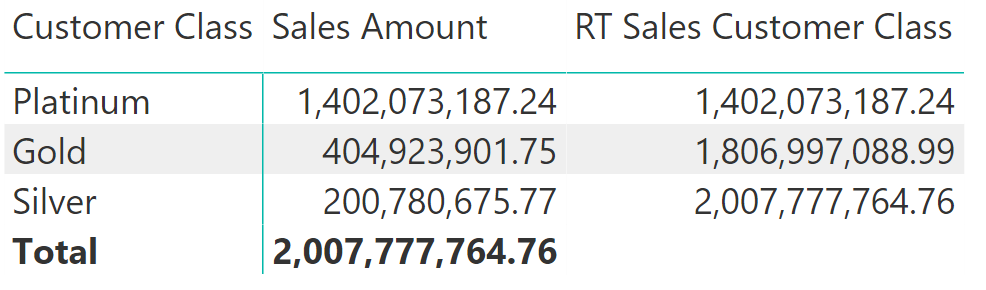A very common calculation in DAX is the year-to-date calculation (YTD), which aggregates values from the beginning of the year all the way to a certain date. A simple implementation uses the predefined DATESYTD function:

```Sales YTD :=
CALCULATE (
[Sales Amount],
DATESYTD( 'Date'[Date] )
)
```

For each month, this returns the aggregated value of all sales in that month plus all previous months within the same calendar year:DATESYTD resets every year. If the goal is to sum values over more than one year, then DATESYTD is no longer useful. In that case, the calculation requires an explicit filter in plain DAX.
The computation of the running total requires a filter that retrieves all the dates prior to the current date in the filter context. Here is a simple way to obtain this:

```Sales RT :=
VAR MaxDate = MAX ( 'Date'[Date] ) -- Saves the last visible date
RETURN
CALCULATE (
[Sales Amount],            -- Computes sales amount
'Date'[Date] <= MaxDate,   -- Where date is before the last visible date
ALL ( Date )               -- Removes any other filters from Date
)
```

First, the MaxDate variable saves the last visible date. Then, two CALCULATE filters remove all the filters on the Date table and they replace the filter on the Date column showing all the dates prior to MaxDate.
The figure below shows the difference between year-to-date which resets at year end, and running totals that carry into the new year.If the column used in the relationship between Date and Sales is a DateTime data type, or if the Date table is marked as a date table, then the ALL ( Date ) statement is not required because it is automatically added by the engine. Nevertheless, the pattern includes that filter for clarity’s sake, so that it can be used even if the relationship does not use a DateTime data type column.
A similar technique can show running totals over different attributes and dimensions. In the demo database, customers are clustered into different categories, based on purchase volume: Platinum, Gold and Silver. What if the user wants a running total of sales amounts for their top tier customers, starting at the top with the Platinum category? The running total pattern is a useful technique here, too.
The goal is to obtain the following report: :The DAX code for RT Sales Customer Class uses the very same pattern as for the running total described earlier:

```RT Sales Customer Class :=
VAR CurrentCustomerClass = SELECTEDVALUE ( Customer[Customer Class Number] )
RETURN
CALCULATE (
[Sales Amount],
Customer[Customer Class Number] <= CurrentCustomerClass,
ALL ( Customer[Customer Class] )
)
```

The running total pattern over tables other than Date is useful for scenarios like ABC classification.